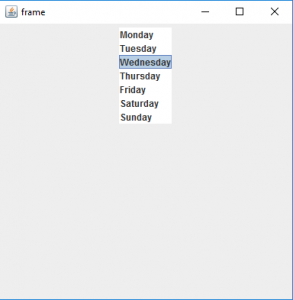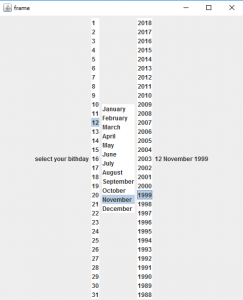Open in App
Not now

# Java Swing | JList with examples

• Last Updated : 16 Apr, 2021

JList is part of Java Swing package . JList is a component that displays a set of Objects and allows the user to select one or more items . JList inherits JComponent class. JList is a easy way to display an array of Vectors .
Constructor for JList are :

1. JList(): creates an empty blank list
2. JList(E [ ] l) : creates an new list with the elements of the array.
3. JList(ListModel d): creates a new list with the specified List Model
4. JList(Vector l) : creates a new list with the elements of the vector

Commonly used methods are :

The Following programs will illustrate the use of JLists
1. Program to create a simple JList

## Java

 `// java Program to create a simple JList` `import` `java.awt.event.*;` `import` `java.awt.*;` `import` `javax.swing.*;` `class` `solve ``extends` `JFrame ` `{` `    `  `    ``//frame` `    ``static` `JFrame f;` `    `  `    ``//lists` `    ``static` `JList b;` ` `    `    ``//main class` `    ``public` `static` `void` `main(String[] args)` `    ``{` `        ``//create a new frame ` `        ``f = ``new` `JFrame(``"frame"``);` `        `  `        ``//create a object` `        ``solve s=``new` `solve();` `      `  `        ``//create a panel` `        ``JPanel p =``new` `JPanel();` `        `  `        ``//create a new label` `        ``JLabel l= ``new` `JLabel(``"select the day of the week"``);`   `        ``//String array to store weekdays` `        ``String week[]= { ``"Monday"``,``"Tuesday"``,``"Wednesday"``,` `                         ``"Thursday"``,``"Friday"``,``"Saturday"``,``"Sunday"``};` `        `  `        ``//create list` `        ``b= ``new` `JList(week);` `        `  `        ``//set a selected index` `        ``b.setSelectedIndex(``2``);` `        `  `        ``//add list to panel` `        ``p.add(b);` ` `  `        ``f.add(p);` `        `  `        ``//set the size of frame` `        ``f.setSize(``400``,``400``);` `         `  `        ``f.show();` `    ``}` `    `  `    `  `}`

Output :2. Program to create a list and add itemListener to it (program to select your birthday using lists) .

## Java

 `// java Program to create a list and add itemListener to it` `// (program to select your birthday using lists) .` `import` `javax.swing.event.*;` `import` `java.awt.*;` `import` `javax.swing.*;` `class` `solve ``extends` `JFrame ``implements` `ListSelectionListener` `{` `    `  `    ``//frame` `    ``static` `JFrame f;` `    `  `    ``//lists` `    ``static` `JList b,b1,b2;` `    `  `    ``//label` `    ``static` `JLabel l1;` ` `    `    ``//main class` `    ``public` `static` `void` `main(String[] args)` `    ``{` `        ``//create a new frame ` `        ``f = ``new` `JFrame(``"frame"``);` `        `  `        ``//create a object` `        ``solve s=``new` `solve();` `      `  `        ``//create a panel` `        ``JPanel p =``new` `JPanel();` `        `  `        ``//create a new label` `        ``JLabel l= ``new` `JLabel(``"select your birthday"``);` `        ``l1= ``new` `JLabel();`   `        ``//String array to store weekdays` `        ``String month[]= { ``"January"``, ``"February"``, ``"March"``,` `        ``"April"``, ``"May"``, ``"June"``, ``"July"``, ``"August"``, ` `        ``"September"``, ``"October"``, ``"November"``, ``"December"``};` `        `  `        ``//create a array for months and year ` `        ``String date[]=``new` `String[``31``],year[]=``new` `String[``31``];` `        `  `        ``//add month number and year to list` `        ``for``(``int` `i=``0``;i<``31``;i++)` `        ``{` `            ``date[i]=``""``+(``int``)(i+``1``);` `            ``year[i]=``""``+(``int``)(``2018``-i);` `        ``}` `        `  `        ``//create lists` `        ``b= ``new` `JList(date);` `        ``b1= ``new` `JList(month);` `        ``b2= ``new` `JList(year);` `        `  `        ``//set a selected index` `        ``b.setSelectedIndex(``2``);` `        ``b1.setSelectedIndex(``1``);` `        ``b2.setSelectedIndex(``2``);` `        `  `        ``l1.setText(b.getSelectedValue()+``" "``+b1.getSelectedValue()` `                              ``+``" "``+b2.getSelectedValue());` `        `  `        ``//add item listener` `        ``b.addListSelectionListener(s);` `        ``b1.addListSelectionListener(s);` `        ``b2.addListSelectionListener(s);` `        `  `        ``//add list to panel` `        ``p.add(l);` `        ``p.add(b);` `        ``p.add(b1);` `        ``p.add(b2);` `        ``p.add(l1);` ` `  `        ``f.add(p);` `        `  `        ``//set the size of frame` `        ``f.setSize(``500``,``600``);` `         `  `        ``f.show();` `    ``}` `    ``public` `void` `valueChanged(ListSelectionEvent e)` `    ``{` `        ``//set the text of the label to the selected value of lists` `        ``l1.setText(b.getSelectedValue()+``" "``+b1.getSelectedValue()` `                              ``+``" "``+b2.getSelectedValue());` `        `  `    ``}` `    `  `    `  `}`

Output :Note : The above programs might not run in an Online compiler please use an Offline IDE

My Personal Notes arrow_drop_up
Related Articles